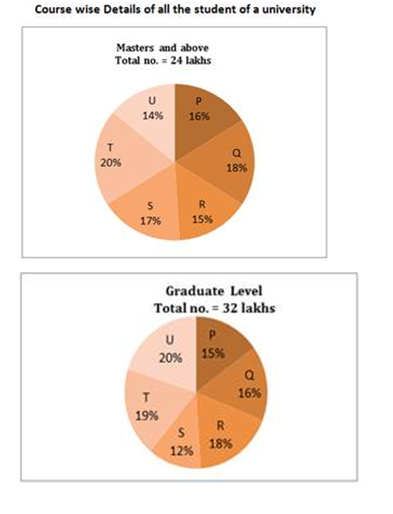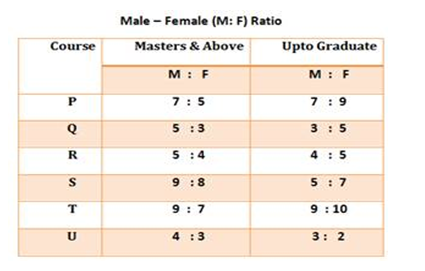# “20-20” Quantitative Aptitude | Crack SBI Clerk / IDBI Executive 2018 Day-52

Dear Friends, SBI Clerk 2018 Notification has been released we hope you all have started your preparation. Here we have started New Series of Practice Materials specially for SBI Clerk / IDBI Executive 2018 . Aspirants those who are preparing for the exams can use this “20-20” Quantitative Aptitude Questions.

[WpProQuiz 1637]

Click “Start Quiz” to attend these Questions and view Solutions

Click Here for SBI Clerk Prelims and Mains 2018 – Full Length Mock Test

Directions (Q. 1-5): What value should come in place of question mark (?) in the following questions?

1. √1024 ÷ 42 × 7.5 + 63 + 279 =?
1. 510
2. 380
3. 260
4. 475
5. None of these
1. (4 × 2)4 ÷ (16 × 4)2 × (32 × 16)5 = 8?
1. 10
2. 6
3. 12
4. 15
5. None of these
1. (5/3) of? – (2/3) of (12/17) of 3162 = 42 % of 850
1. 783
2. 857
3. 1107
4. 685
5. None of these
1. 25 % of x – 1664 ÷ 13 + 222 ÷ 6 = 67
1. 968
2. 745
3. 529
4. 632
5. None of these
1. √x × 13 – 26 % of 2650 – 12 × 28 = 15
1. 80
2. 3600
3. 60
4. 6400
5. None of these

Directions (Q. 6 – 10): What value should come in place of (?) in the following series?

1. 809, 811, 820, 848, 913, ?
1. 1185
2. 934
3. 1257
4. 1039
5. None of these
1. 87, 87, 83, 99, 63, ?
1. 98
2. 127
3. 143
4. 156
5. None of these
1. 294624, 24552, 2232, 248, ?
1. 72
2. 64.6
3. 49.6
4. 98
5. None of these
1. 856, 213, 52.25, ? , 2.015625
1. 32.50
2. 24.075
3. 12.0625
4. 38.0225
5. None of these
1. 727, 513, 390, 328, ?
1. 303
2. 286
3. 212
4. 311
5. None of these

1. The ratio between cost price of three articles A, B and C is 2:3:4 while the profit percentage earned in these articles is in the ratio of 5:4:3 and profit earned on article C is Rs 300. If the cost price of article A is Rs 1000, find the selling price of article A?
1. 2500
2. 2180
3. 1250
4. 600
5. 1500
1. A and B invest in the ratio of 25:17. If they divide 40% of the profit equally and rest in the proportion of their investments, then find B’s profit if A’s profit is Rs 1950.
1. 1650
2. 1550
3. 4500
4. 5600
5. 1420
1. A shopkeeper sells a transistor at 15% above its cost price. If he had bought it 5% more than what he paid for it and sold it for Rs 6 more, he would have gained 10%. The cost price of the transistor is
1. 800
2. 1000
3. 1200
4. 1400
5. None of these
1. The present age of Ravi’s father is four times Ravi’s present age. Five years back, Ravi’s father was seven times as old as Ravi was at that time. What is the present age of Ravi’s father?
1. 84
2. 70
3. 40
4. 35
5. None of these
1. The length and breadth of a rectangular piece of land are in the ratio of 5: 3. The owner spent Rs 3000 for surrounding it from all the sides at the rate of Rs 7.50 per meter. The difference between length and breadth is:
1. 50m
2. 100m
3. 200m
4. 150m
5. None of these

Directions (Q. 16 – 20): Study the following pie-charts and table to answer these questions.1. What is the difference between the Masters male student and Graduate male student from Course ‘P’?
1. 24000
2. 14000
3. 28000
4. 36000
5. None of these
1. What is the ratio of the Masters female student of Course ‘T’ to Graduate female student of Course ‘S’?
1. 7 : 5
2. 5 : 7
3. 16 : 15
4. 15 : 16
5. None of these
1. The Masters female student of Course ‘R’ is what per cent of the Graduate female student of that Course?
1. 40
2. 62.5
3. 50
4. 52.5
5. None of these
1. The Graduate male student of Course ‘R’ is what per cent of the Graduate male student of course ‘S’?
1. 180%
2. 120%
3. 110%
4. 190%
5. None of these
1. What is the ratio of the Masters male student of Course ‘T’ to the Graduate female student of that Course?
1. 28 : 35
2. 35 : 28
3. 32 : 45
4. 45 : 32
5. None of these

Directions (Q. 1-5):

(32/16)*(15/2) + 216 + 279 = x

15 + 216 + 279 = x

X = 510

84 ÷ 642 × 5125 = 8x

84 ÷ 84 × 815 = 8x

84-4+15 = 8x

815 = 8x

X = 15

(5x/3) – (2/3) * (12/17) * 3162 = (42/100) * 850

(5x/3) – 1488 = 357

(5x/3)  = 1845

X = (1845*3)/5 = 1107

25 % of x – 1664 ÷ 13 + 222 ÷ 6 = 67

(25/100)*x – 128 + 37 =67

(25/100)*x = 67 + 128 -37

(25/100)*x = 158

X = (158*100)/25

X = 632

√x × 13 – 26 % of 2650 – 12 × 28 = 15

√x × 13 – (26/100)*2650 – 336 = 15

√x × 13 – 689 – 336 = 15

√x × 13 = 15 +689 + 336

√x × 13 = 1040

√x = 1040/13 = 80

X = 802 = 6400

Directions (Q. 6 – 10):

The difference is, 13+1, 23+1, 33+1, 43+1, 53+1,..

The pattern is, +02, -22, +42, -62, +82,..

The pattern is, ÷ 12, ÷ 11, ÷ 9, ÷ 5,..

The pattern is, ÷ 4 -1

The pattern is, -(63 – 2), -(53 – 2), -(43 – 2), -(33 – 2),..

Cost price article C = 1000*4/2= Rs 2000

Profit percentage earned on article C= 300*100/2000= 15%

Percentage profit earned in article A= 15*5/3= 25%

Profit earned in article A= 1000*25/100= Rs 250

Selling price of article A= 1000+250= Rs 1250

Let the total profit be x

Then

A’s profit= 0.2x+0.6x*25/42

1950= 0.2x (1+75/42)

X= 1950*42/0.2*117= Rs 3500

B’s profit= 3500-1950= Rs 1550

Let the cost of the transistor be x

SP = 115/100*x

New CP = x* 105/100= 105x/100

New SP= 115x/100+ 6=105x/100*110/100

=>115x/100-231x/200= -6

=>x=1200

Let the present age of Ravi be x

Present age of Ravi’s father= 4x

Now 5 yr ago

Ravi’s father’s age= 7 * Ravi’s age

= 4x-5=7(x-5)

= x= 10

Ravi’s Father’s age= 4*10= 40 years.

Perimeter of the field = 3000/7.50= 400m

So,

2(5x + 3x) = 400 => x = 25

So, length = 125 m & breadth = 75 m

= (125-75)m = 50m

Directions (Q. 16 – 20):

Masters Male Student from Course ‘P’

= 24*(16/100)*(7/12) lakh

= 2,24,000

Graduate male student from Course ‘P’

= 32*(15/100)*(7/16) lakh

= 210000
Difference = 224000- 210000 = 14000

Masters female student of course T

= 24*(20/100)*(7/16) lakh

Graduate female student of course S

= 32*(12/100)*(7/12) lakh
Required ratio = [24*(20/100)*(7/16)] : [32*(12/100)*(7/12)]

= 210 : 224

= 15 : 16

Masters female student of course R

= 24*(15/100)*(4/9) = 1.6 lakh
Graduate female student of course R

= 32*(18/100)*(5/9) = 3.2 lakh

Required % =(1.6/3.2)*100 = 50 %

Graduate male student of course R

= 32*(18/100)*(4/9)

Graduate male student of course S

= 32 *(12/100)*(5/12)

Required % = {[32*(18/100)*(4/9)]/[ 32 *(12/100)*(5/12)]}*100

= 160 %

Masters male student of course T

= 24*(20/100)*(9/16)

Graduate female student of course T

= 32*(19/100)*(10/19)

Required ratio is,

= [24*(20/100)*(9/16)] : [32*(19/100)*(10/19)]

= 27 : 32

Daily Practice Test Schedule | Good Luck

 Topic Daily Publishing Time Daily News Papers & Editorials 8.00 AM Current Affairs Quiz 9.00 AM Logical Reasoning 10.00 AM Quantitative Aptitude “20-20” 11.00 AM Vocabulary (Based on The Hindu) 12.00 PM Static GK Quiz 1.00 PM English Language “20-20” 2.00 PM Banking Awareness Quiz 3.00 PM Reasoning Puzzles & Seating 4.00 PM Daily Current Affairs Updates 5.00 PM Data Interpretation / Application Sums (Topic Wise) 6.00 PM Reasoning Ability “20-20” 7.00 PM English Language (New Pattern Questions) 8.00 PM General / Financial Awareness Quiz 9.00 PM